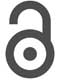## Volume 3 - Issue 4

Short CommunicationCreative Commons, CC-BY

# Effect of Wire Tension on Rate of Weight Bearing in Ilizarov Apparatus

*Corresponding author: Mohammad Reza Effatparvar, MSc of Biomedical Engineering, Department of Biomedical Engineering, Faculty of Engineering, University of Isfahan, Kargar st, Tehran, Iran.

Received: June 10, 2019; Published: June 20, 2019

#### Abstract

K-wires play an important role in stability of Ilizarov fixator. In this fixation system, wires are tensioned for bearing patient weight. In this study, measuring of vertical bending of k-wire would show the effect of horizontal tension of wire. Russian Ilizarov K-wires were inserted into artificial tibia sawbones and fixed on the ring. Then, after wire tensions in range of 70kg to 130kg by tensioner device and vertical forces of 40kg to 100kg by bending test device, vertical displacements were measured. 49 data about to wire displacements are recorded. in the minimum horizontal tension (P) (70 kg) and maximum vertical loading (F) (100 kg), the rate of vertical displacement (Y) received to the highest amount (0.837 cm), but in condition that P was maximum (130 kg) and F was minimum (40 kg) the amount of Y got to the lowest point (0.112). Regarding to the obtain data, effect of k-wire tensile on decreasing the vertical displacement and prevent from k-wire cutting is obviously Figure out. More tension is better way to earning stability. This should be noted; the maximum amount of tension is the half of yield point.

Keywords: K-Wire; Ilizarov; Tension; Vertical Displacement

#### Introduction

Krishner wire or k-wire is a pin which used in orthopedic surgeries. One of the main applications of these wires is in Ilizarov external fixation. In this method wire is inserted into bone from one side and extracted from other side . The wire must be fixed on the ring of Ilizarov frame from one end by wire fixation bolt and tensioned from another end by tensioner device . The fixation strength has a direct relation to the tension of the wires [3,4]. This performance is like to the bicycle spokes tension for increasing weight bearing. Not enough wire tension will lead to multiple bending and finally wire cutting . Basically, the tension rate of the wires depends on patient weight [6,7]. Standard range of tension for stainless steel wire with diameter of 1.8 mm is 70kg to 130 kg . In this experiment effect of tension on vertical displacement of wire will investigated.

#### Material and Method

An artificial tibia sawbones with diameter of 40mm was used as a bone simulator. Stainless steel k-wires of Russian Ilizarov scientific center with diameter of 1.8mm were inserted into sawbones by drill. Then wire fixed and locked from one side by wire fixation bolt on the stainless-steel ring of Indian Pitkar Company with diameter of 160 mm. In next step, wire was tensioned by Russian Ilizarov scientific center tensioner device from 70kg to 130kg with 10kg intervals. After applying each step of the tension, this set from the center was located at the site of the Gotech AI-7000M bending test device. The vertical force on wire, which simulates the force of the body weight was inserted from 40kg to 100kg with 10kh intervals. The process of test is showed below. In process, wire is tensioned by amount of P then vertical force of F is applied and displacement(y) is recorded (Figure 1).

#### Results and Discussion

After conducting several experiments, k-wire vertical displacements (y) were recorded as shown in Table 1. In the table above, the values of F mean the tensions entered the wires in kilograms and the values of P are the weights entered vertically on the wires in kilograms. Each cell inside the table show the amount of displacements or the pin’s bending, in cm. For example, the bending of the wire in the case of 130 kg tension (F) and 100kg vertical force (p) is 0.396 cm. The highest vertical displacement of 837.0 cm is related to horizontal stretching of 70 kg and vertical loading of 100 kg.

The axial tensile force on the k-wire in Ilizarov external fixator is the most basic method of stability. The experiments in this paper have clear results regarding the need for horizontal tension in the wire to increase the apparatus weight bearing capacity. It should be noted that, more k-wires usage in one ring will lead to more stability and lesser displacement. As can be seen from the table’s results, in each raw, from left to right, and in each column from down to up the numbers are incremental. In this test, while the axial tensile force increases from 70 to 130 kg the rate of bending or vertical displacement (Y) decreases. But it should not be forgotten that the hypertension of k-wire because of diameter change will result inversely. When the tension of k-wire (p) be close to the loading amount (F), vertical displacement will be less. But if the bearing of wire gets more than tension rate, the amount of vertical displacement will increase linearly . Most of the time, k-wire cutting is Due to increased fatigue rates because of multiple bends.

#### Conclusion

Finally, the results show, the maximum displacement is occurred in minimum tension and maximum bearing, In the case of a k-wire usage for a person with a high weight, tensile force should be increased in proportion to the weight of the patient.